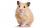# Examples of area of plane shapes

1. Perimeter to areaCalculate the area of a circle with the perimeter 15 meters.
2. Diagonals of diamondFind the area and circumference of the diamond ABCD with 15m and 11m diagonals.
3. Trapezoid - central medianThe central median divides the trapezoid into two smaller trapezoids. Determines the ratio of their contents.
4. Rectangular trapezoidThe rectangular trapezoid ABCD is: /AB/ = /BC/ = /AC/. The length of the median is 6 cm. Calculate the circumference and area of a trapezoid.
5. Diameter to areaFind the area of a circle whose diameter is 26cm.
6. TrapeziumThe lengths of a parallel sides of a trapezium are (2x+3) and (x+8) and the distance between them is (x+4). if the area of the trapezium is 590 , find the value of x.
7. Triangle ABC v2Area of the triangle is 12 cm square. Angle ACB = 30º , AC = (x + 2) cm, BC = x cm. Calculate the value of x.
8. PentagonCalculate the length of side, circumference and area of a regular pentagon, which is inscribed in a circle with radius r = 6 cm.
9. 2d shapeCalculate the content of a shape in which an arbitrary point is not more than 3 cm from the segment AB. The length of the segment AB is 5 cm.
10. Rectangle 3-4-5The sides of the rectangle are in a ratio of 3:4. The length of the rectangle diagonal is 20 cm. Calculate the content of the rectangle.
11. Perimeter from areaWhat is the perimeter of the square if its content is 64 cm2?
12. Hamster cageRyan keeps his hamster cage on his dresser. The area of the top of Ryan's dresser is 1 2\3 as large as the area of the bottom of his hamster cage. The area of the dresser top is 960 square inches. How many square inches of his dresser top are not covered
13. Cuboid and eq2Calculate the volume of cuboid with square base and height 6 cm if the surface area is 48 cm2.
14. Diagonals of a rhombusOne of the diagonals of a rhombus is twice as the other. If the sum of the lengths of the diagonals is 24, find the area of the rhombus.
15. Right triangle eq2Hypotenuse of a right triangle is 9 cm longer than one leg and 8 cm longer than the second leg. Determine the circumference and area of a triangle.
16. Rhombus and diagonalsThe a rhombus area is 150 cm2 and the ratio of the diagonals is 3:4. Calculate the length of its height.
17. Trapezium 2Trapezium has an area of 24 square cms. How many different trapeziums can be formed ?
18. Square vs rectangleSquare and rectangle have the same area contents. The length of the rectangle is 9 greater and width 6 less than side of the square. Calculate the side of a square.
19. Trapezoid MO-5-Z8ABCD is a trapezoid that lime segment CE divided into a triangle and parallelogram as shown. Point F is the midpoint of CE, DF line passes through the center of the segment BE and the area of the triangle CDE is 3 cm2. Determine the area of the trapezoid
20. HypotenuseCalculate the length of the hypotenuse of a right triangle if the length of one leg is 4 cm and its content area is 16 square centimeters.

Do you have an interesting mathematical word problem that you can't solve it? Submit math problem, and we can try to solve it.

We will send a solution to your e-mail address. Solved examples are also published here. Please enter the e-mail correctly and check whether you don't have a full mailbox.

Please do not submit problems from current active competitions such as Mathematical Olympiad, correspondence seminars etc...